## How to import questions via plain text

It's now easier than ever to import questions into BetterExaminations. Importing via plain text allows you to import questions into your question bank by writing your questions in a very simple, plain-text format.

This means that you can quickly write questions inside any document editor, and then bulk import them into your question bank in a matter of seconds.

## Supported question types

Currently, our plain text import tool supports the following question types

• Multiple Choice (Standard)
• True/False
• Essay with rich text

As always, we will continue to expand our supported question types for this tool in the future.

## Step-by-step guide

To start importing questions, navigate to your question bank and select 'Import questions'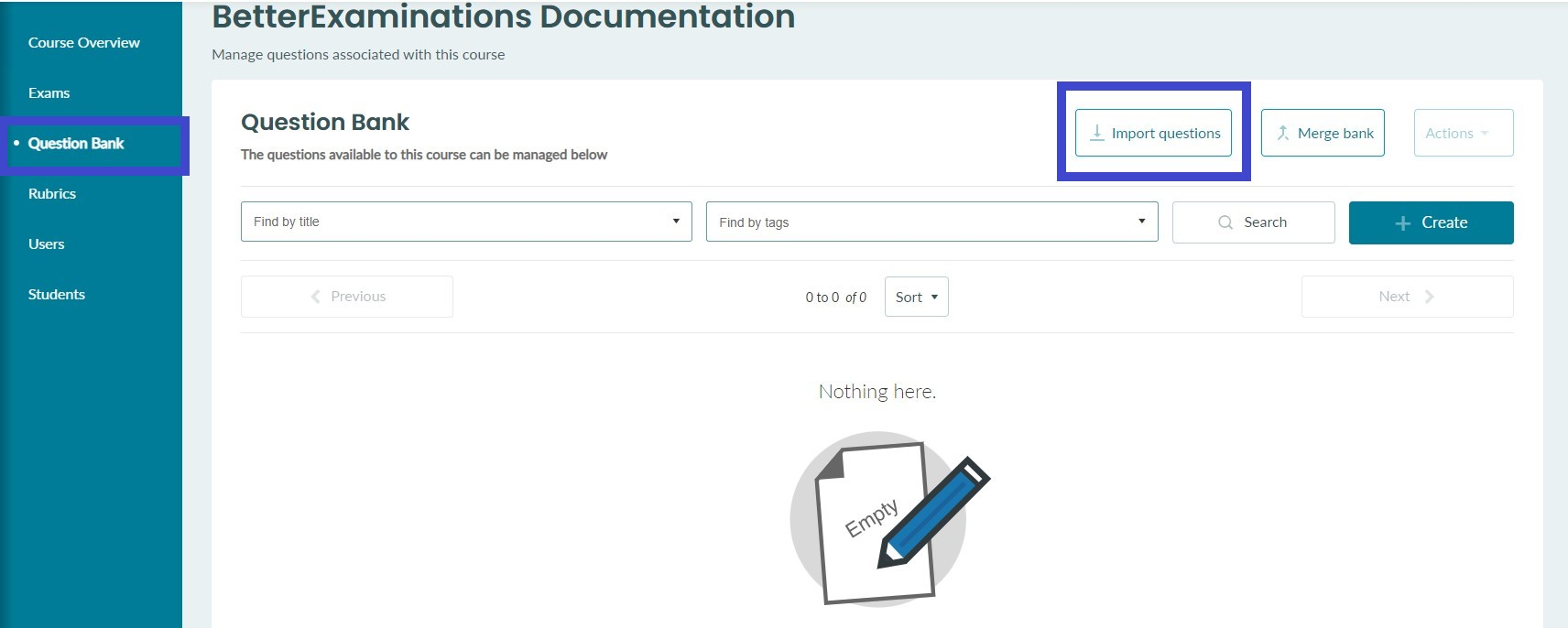Select the 'Plain text' option from the dropdown menu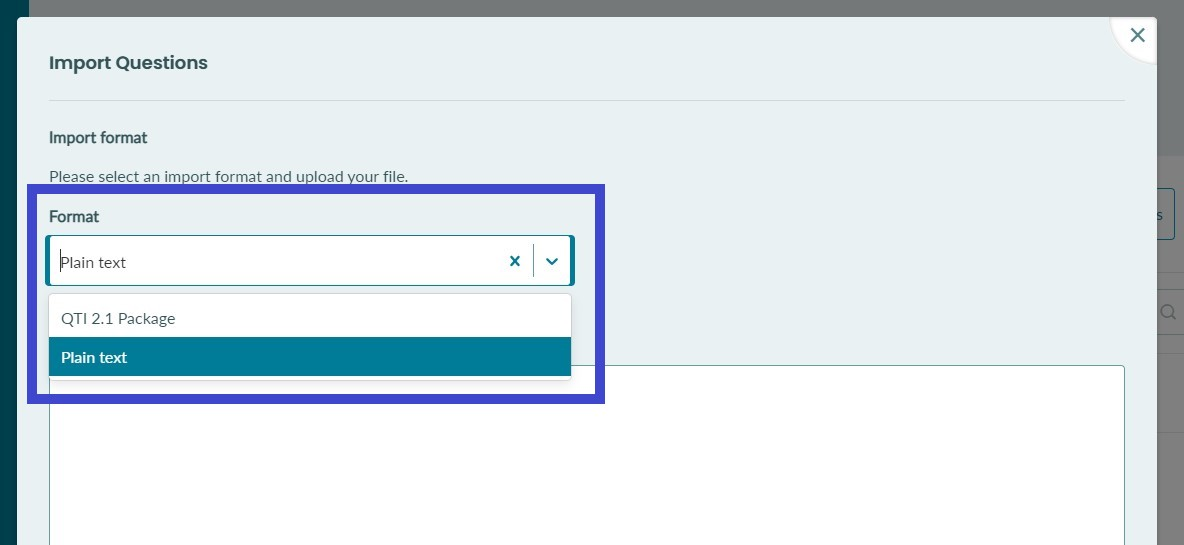You can then start typing your questions into the text box OR copy and paste them from a word document. There are four different question types supported.

### Importing Multiple Choice Standard Questions

Multiple choice questions should be written in the following format

MCQ
[TITLE] Q1 - BE10001
[STEM] What is the capital of Ireland? :
Paris
New York
*Dublin
London

Let's examine the makeup of our MCQ question below

MCQ   `<---- Question type`
[TITLE] BE10001 `<---- Question title - This is how you idenfiy the question - This is NOT shared with the student`
[STEM] What is the capital of Ireland? :   `<-- Question stem and points - The stem is the part of the question the student will see`
Paris   `<---- Wrong answer`
New York    `<---- Wrong answer`
*Dublin    `<---- Correct answer marked with an asterisk`
London    `<---- Wrong answer`

Rules for MCQ format

(1) The question should start with a single line containing the text `MCQ`

(2) The next line should contain the question title. It should begin with `[TITLE]`. The title is how you identify the question within the exam editor and question bank. It is common practice to include a unique ID here so that you can easily identify your question. This will NOT be shared with the student.

(3) The next line should contain the question stem. It should begin with `[STEM]`. This is the question text that will be shown to students in the exam.

(4) At the end of the `[STEM]` line, you can define the points the question is worth. This is done by adding a colon followed by square brackets containing the number of points the question is worth. For example, a question worth five points would look like this

`[STEM] This is my questionstem :`

Note: If you do not specify any points at the end of the question, the correct answer will be, by default, worth 1 point.

Note: If you do not specify any points OR correct answer the question will be, by default, worth 0 points.

(4) Each new line after that should contain one of the answer options.

Paris   `<---- Wrong answer`
New York    `<---- Wrong answer`
*Dublin    `<---- Correct answer marked with an asterisk`
London    `<---- Wrong answer`

So, let's see what that would look like in the import tool. Remember, you don't have to type the questions directly into the import tool. You can write them up in any text editor and paste them in.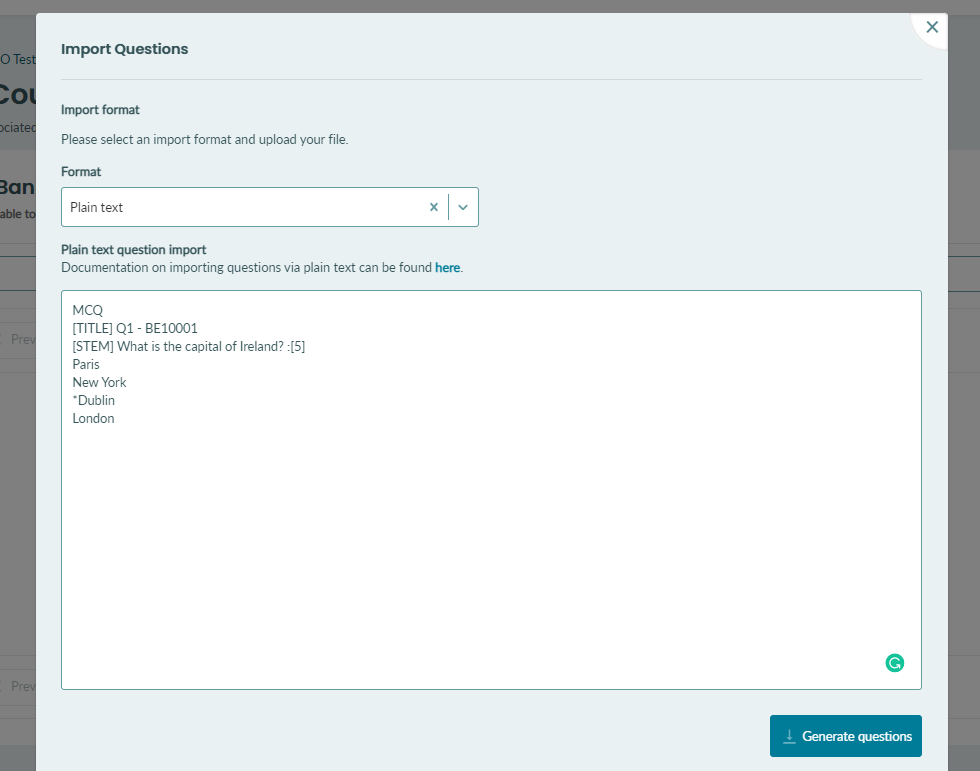Once your question is in the text box, click the 'Generate questions' button at the bottom right of the text box.

You should now see a table appear beneath the button. It should contain your new question title, the question type (MCQ), and the points the question is worth.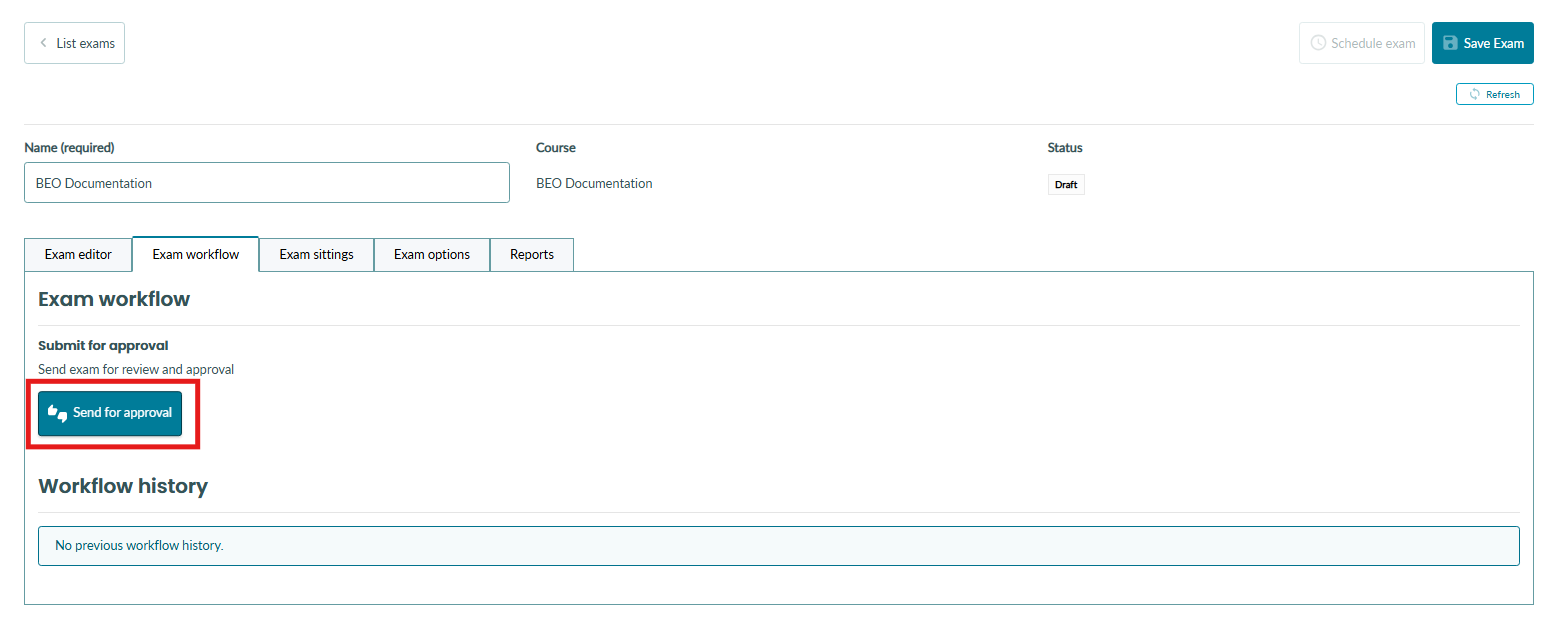You can generate multiple MCQ questions in one go. Simply separate each question with a new line. See the example below where we have generated 3 questions.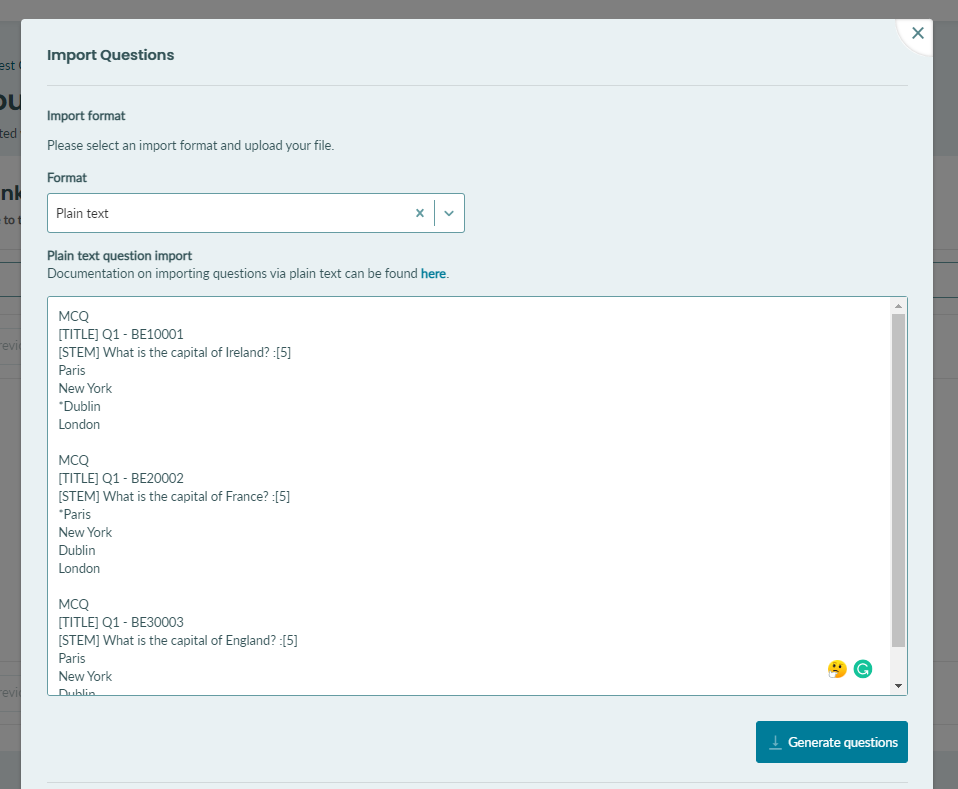Once you're ready to import your questions, simply select the 'Import questions' button below the table.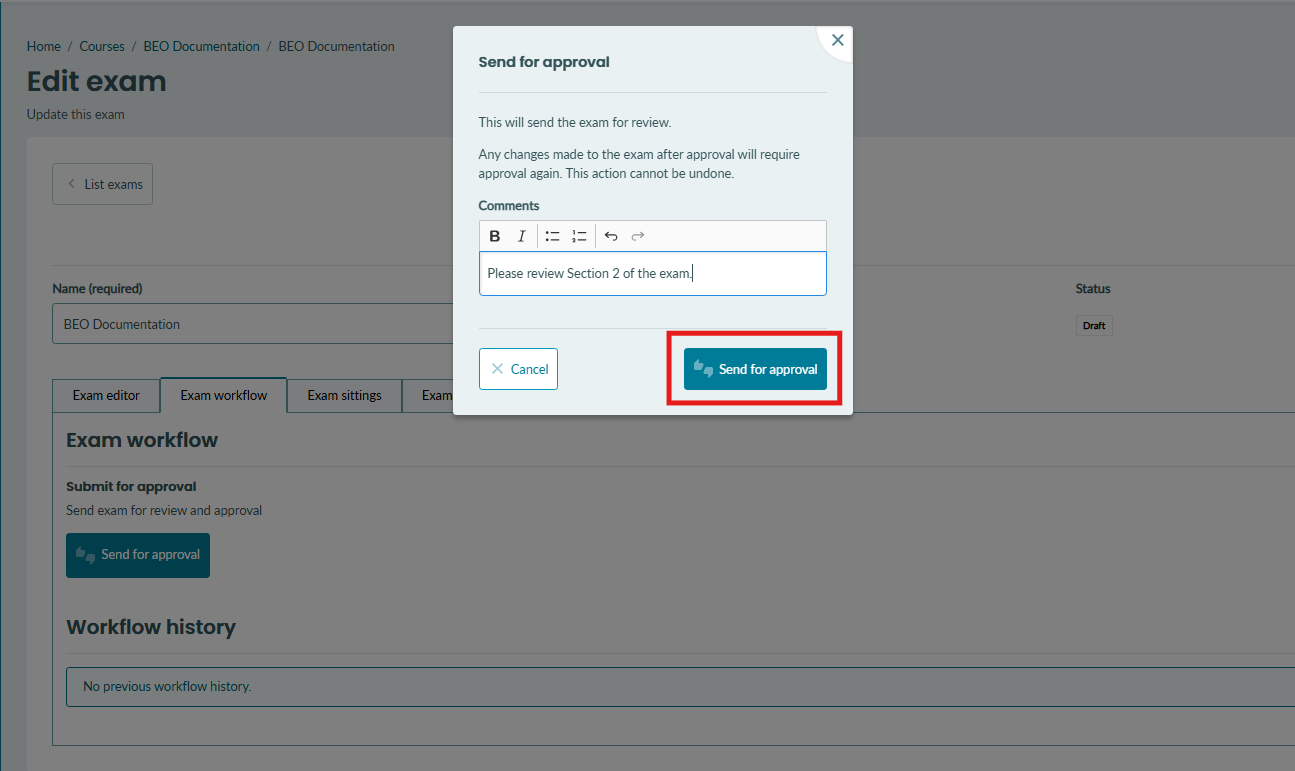Once the import has been completed, you should see your questions appear in your question bank.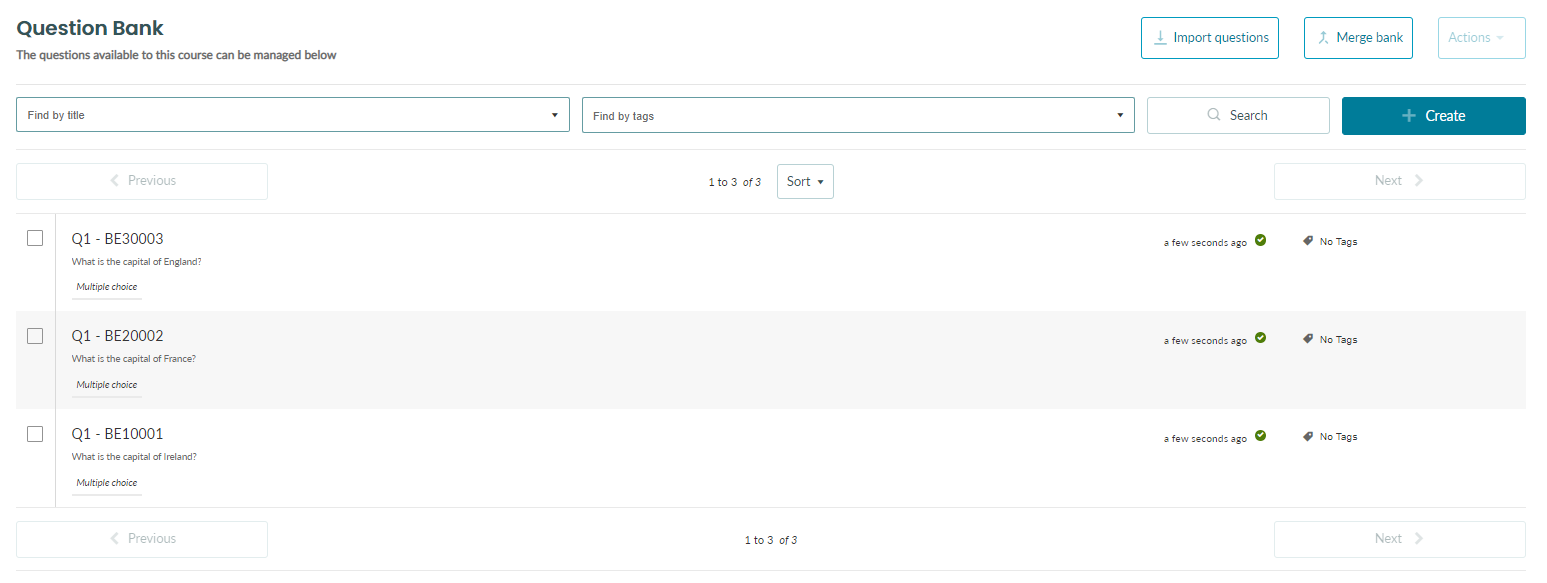### Importing Multiple Choice - Multiple Response Questions

The procedure for importing Multiple Choice - Multiple Response questions is very similar to that of standard multiple-choice questions.

Multiple Choice - Multiple Response questions should be written in the following format

MA
[TITLE] Q1 - BE100001
[STEM] Select all prime numbers :
*3
*5
6
*7

Let's examine the makeup of our Multiple Choice - Multiple Response question below

MA  `<---- Question type`
[TITLE] BE10001 `<---- Question title - This is how you idenfiy the question - This is NOT shared with the student`
[STEM] Select all prime numbers :   `<----- Question stem and points - The stem is the part of the question the student will see `
*3    `<---- One of the correct answers marked with an asterisk`
*5   `<---- Another one of the correct answers marked with an asterisk`
6   `<---- Wrong answer`
*7   `<---- Another one of the correct answers marked with an asterisk`
8   `<---- Wrong answer`

Rules for Multiple Choice - Multiple Response format

(1) The question should start with a single line containing the text `MA`

(2) The next line should contain the question title. It should begin with `[TITLE]`. The title is how you identify the question within the exam editor and question bank. It is common practice to include a unique ID here so that you can easily identify your question. This will NOT be shared with the student.

(3) The next line should contain the question stem. It should begin with `[STEM]`. This is the question text that will be shown to students in the exam.

(4) At the end of the `[STEM]` line, you can define the points the question is worth. This is done by adding a colon followed by square brackets containing the number of points the question is worth. For example, a question worth five points would look like this

`[STEM] This is my question stem :`

Note: If you do not specify any points at the end of the question, the question will be, by default, worth 1 point.

Note: If you do not specify any points OR correct answer the question will be, by default, worth 0 points.

(5) Each new line after that should contain one of the possible answers.

Importing True/False questions

The procedure for importing true/false questions is very similar to that of standard multiple-choice questions.

True/False questions should be written in the following format

TF
[TITLE] Q1 - BE100001
[STEM] Dublin is the capital of Ireland :
*True
False

Let's examine the makeup of our True/False question below

TF   `<---- Question type`
[TITLE] BE10001 `<---- Question title - This is how you idenfiy the question - This is NOT shared with the student`
[STEM] Dublin is the capital of Ireland :   `<----- Question stem and points - The stem is the part of the question the student will see `
*True    `<---- Correct answer marked with an asterisk`
False   `<---- Wrong answer`

Rules for True/False format

(1) The question should start with a single line containing the text `TF`

(2) The next line should contain the question title. It should begin with `[TITLE]`. The title is how you identify the question within the exam editor and question bank. It is common practice to include a unique ID here so that you can easily identify your question. This will NOT be shared with the student.

(3) The next line should contain the question stem. It should begin with `[STEM]`. This is the question text that will be shown to students in the exam.

(4) At the end of the `[STEM]` line, you can define the points the question is worth. This is done by adding a colon followed by square brackets containing the number of points the question is worth. For example, a question worth five points would look like this

`[STEM] This is my question stem :`

(5) The next line should contain the word `True`. If this statement is true, an asterisk (*) should be added before the word. `*True`

(6) The next line should contain the word `False`. If this statement is false, an asterisk (*) should be added before the word. `*False`

Importing Essay Questions

Essay questions should be written in the following format

ES
[TITLE] Q1 - BE100001
[STEM] Write on the possibility of an alien invasion :

Let's examine the makeup of our Essay question below

ES   `<---- Question type`
[TITLE] BE10001 `<---- Question title - This is how you idenfiy the question - This is NOT shared with the student`
[STEM] Write on the possibility of an alien invasion :   `<----- Question stem and points - The stem is the part of the question the student will see `

Rules for Essay format

(1) The question should start with a single line containing the text `ES`

2) The next line should contain the question title. It should begin with `[TITLE]`. The title is how you identify the question within the exam editor and question bank. It is common practice to include a unique ID here so that you can easily identify your question. This will NOT be shared with the student.

(3) The next line should contain the question stem. It should begin with `[STEM]`. This is the question text that will be shown to students in the exam.

(4) At the end of the `[STEM]` line, you can define the points the question is worth. This is done by adding a colon followed by square brackets containing the number of points the question is worth. For example, a question worth five points would look like this

`[STEM] This is my question stem :`

Note: If you do not specify any points at the end of the question, the question will be, by default, worth 1 point.

Note: If you do not specify any points OR correct answer the question will be, by default, worth 0 points.

Note regarding question formatting

Currently, there is a limitation that means question titles and stems are restricted to one paragraph and rich text formatting is not supported. It is not possible to add new lines in the middle of a question title or stem. Please make sure to check your imported questions to ensure that all content is present.